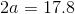## Example Questions

### Example Question #1 : Pyramids

A square pyramid has a volume of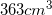and a height of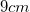. What is the perimeter of the base of the pyramid?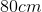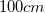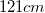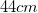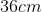Explanation:

The formula for the volume of a pyramid is: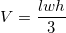We know that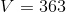and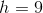; however, this still leaves us with two variables in the equation:and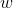. By definition, a square pyramid's base has sides of equal length, meaning thatandare the same. Therefore, we can substitute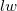for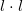, or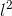.

This gives us a new equation of: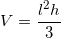We then plug in the variables we know: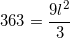Multiply both sides by 3: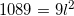Divide both sides by 9: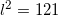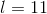Therefore, we now know that the length of the pyramid's base is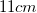. The question, however, asks for the perimeter of the pyramid's base. Since all of the sides of the base are the same, they must all be. So we multiply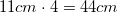. Therefore, the perimeter of the pyramid's base is.

### Example Question #2 : Pyramids

A square pyramid has a slant height of 8. The edge of a face is 12. What is the measure of an edge of its base?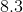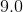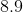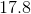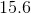Explanation:

In this kind of a problem, it's helpful to draw out all information that's given.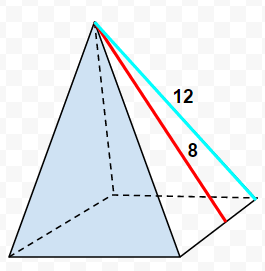Because the triangular faces of a pyramid are isosceles triangles, the slant height can be seen to divide it into two right triangles. The goal is to find the measure of the edge of the square base. This quickly becomes a problem revolving around the use of the Pythagorean theorem.

If we take the measures 12 and 8 to be the hypotenuse and one of the legs, respectively, we can use the Pythagoream theorem to solve for the "base leg." Then, realizing that this measure if only half of the the total length of the square base edge, that value must be multiplied by 2.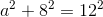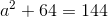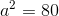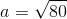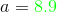Therefore,